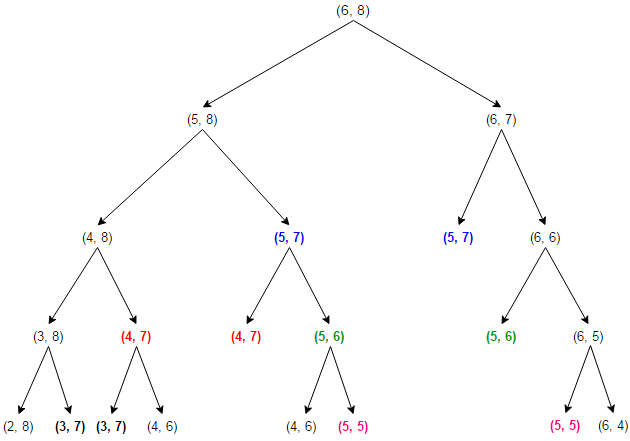# Shortest Common Supersequence | Introduction & SCS Length

The shortest common supersequence (SCS) is the problem of finding the shortest supersequence Z of given sequences X and Y such that both X & Y are subsequences of Z.

For example, consider the two following sequences X and Y

X: ABCBDAB
Y: BDCABA

The length of SCS is 9
SCS are ABCBDCABA, ABDCABDAB and ABDCBDABA

The SCS problem has an optimal substructure. That means the problem can be broken down into smaller, simple “subproblems”, which can be broken down into yet simpler subproblems, and so on, until, finally, the solution becomes trivial.

1. Let us consider two sequences X and Y of length m and n respectively that both end in the same element.

To find their SCS, we shorten each sequence by removing the last element, find the SCS of the shortened sequences, and to that SCS append the removed element. So we can say that

SCS(X[1..m], Y[1..n]) = SCS(X[1..m-1], Y[1..n-1]) + X[m]   if X[m] = Y[n]

For example,

SCS(‘ABCBDA’, ‘BDCABA’) = SCS(‘ABCBD’, ‘BDCAB’) + ‘A’
SCS(‘ABCBDAB’, ‘BDCAB’) = SCS(‘ABCBDA’, ‘BDCA’) + ‘B’

2. Now suppose that the two sequences do not end in the same element.

Then the SCS of X and Y is the shorter of the two sequences SCS(X[1..m-1], Y[1..n]) + X[m] and SCS(X[1..m], Y[1..n-1]) + Y[n]. To understand this property, let’s consider the two following sequences

X: ABCBDAB (n elements)
Y: BDCABA  (m elements)

The SCS of these two sequences either ends with a B (the last element of sequence X) or with A (the last element of sequence Y).

Case 1: If SCS ends with a B, then we add B from sequence X to SCS and the problem reduces to SCS(X[1..m-1], Y[1..n]) + X[m].

Case 2: If SCS does not end with a B, that means it ends with A, then we add A from sequence Y to SCS and the problem reduces to SCS(X[1..m], Y[1..n-1]) + Y[n].

For example,

SCS(‘ABCBD’, ‘BDCAB’) = minimum (SCS(‘ABCB’, ‘BDCAB’) + ‘D’,
SCS(‘ABCBD’, ‘BDCA’) + ‘B’)

Below solution finds the length of shortest common supersequence of sequences X[0..m-1] and Y[0..n-1] recursively by using optimal substructure property of SCS problem.

## C++

Output:

The length of shortest Common supersequence is 9

## Java

Output:

The length of shortest Common supersequence is 9

The worst case time complexity of above solution is O(2(m+n)) and auxiliary space used by the program is O(1). The worst case happens when there is no common character present in X and Y (i.e. SCS length is (m+n)) and each recursive call will end up in two recursive calls.

The SCS problem exhibits overlapping subproblems. Let us consider recursion tree for two sequences of length 6 and 8 whose SCS length is (6+8=14). As we can see, the same sub-problems (highlighted in same color) are getting computed again and again –The problems having optimal substructure & overlapping subproblems can be solved by dynamic programming, in which subproblem solutions are memoized rather than computed again and again. Below is Memoized version that follows the top-down approach, as we first break the problem into subproblems and then calculate and store values.

## C++

Output:

The length of shortest Common supersequence is 9

## Java

Output:

The length of shortest Common supersequence is 9

The time complexity of above solution is O(mn) and auxiliary space used by the program is O(mn).

We can also solve this problem in bottom-up manner. In the bottom-up approach, we calculate the smaller values of SCS(i, j) first, then build larger values from them.

| j                                      (if i == 0)
| i                                      (if j == 0)
SCS[i][j] = | SCS[i-1][j-1] + 1                      (if X[i-1] == Y[j-1])
| min (SCS[i-1][j] + 1, SCS[i][j-1] + 1) (if X[i-1] != Y[j-1])

## C++

Output:

The length of shortest Common supersequence is 9

## Java

Output:

The length of shortest Common supersequence is 9

The time complexity of above solution is O(mn) and auxiliary space used by the program is O(mn). The space complexity of above solution can be improved to O(n) as calculating SCS of a row of the SCS table requires only the solutions to the current row and the previous row.

Exercise:

1. Extend the solution for Printing Shortest Common Supersequence.
2. Write space optimized code for iterative version.(2 votes, average: 5.00 out of 5)Loading...

Please use our online compiler to post code in comments. To contribute, get in touch with us.
Like us? Please spread the word and help us grow. Happy coding 🙂